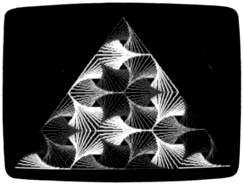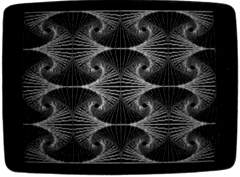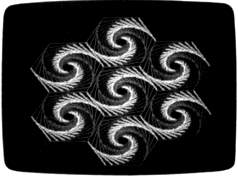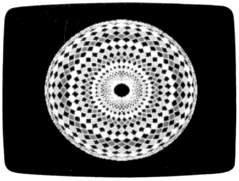`COMPUTE! ISSUE 72 / MAY 1986 / PAGE 86`

MODified Shapes For IBM

Paul W. Carlson

Ever wonder how to use the MOD operator in IBM BASIC? Here's a short explanation of MOD operations that create some stunning graphics displays as well. The programs run on any IBM PC with color/graphics adapter and BASICA or PCjr with Cartridge BASIC.

Though you may never have seen it used, IBM BASIC's MOD operator is a powerful tool for performing certain arithmetic operations. Simply put, MOD gives the integer (whole number) remainder of an integer division. For example, 17 MOD 3 = 2 because 17 divided by 3 equals 5 with a remainder of 2. Likewise, 30 MOD 5 = 0 since 5 divides into 30 evenly with a remainder of zero.
To see some applications of MOD arithmetic, type in, save, and run Programs 1-4 below. Each generates a different geometric display (see photos), using MOD in various ways to simplify the graphics calculations.
Although some dialects of BASIC don't include a MOD operator, the INT function can be used for the same purpose. In Microsoft BASIC, the expression K-INT(K/J)*J gives exactly the same result as the IBM BASIC expression K MOD J. If your computer's BASIC doesn't have MOD but does have a line-drawing command (the Commodore 128 and Atari fall into this category), you may find it interesting to convert the programs to run on your machine.

What Does MOD Do?
One of the most common uses of MOD is to test whether a value is odd or even. The expression X MOD 2 yields a 1 if X is odd, or 0 if X is even. Program 2 uses MOD for this purpose in line 20, branching to line 40 only when the variables I and J are both odd or even.
Another common use of MOD is to make a variable equal to a repeating sequence of consecutive integers. Consider these statements:

10 C=0
20 C=C MOD 3+1
30 PRINT C
40 GOTO 20

In this case the variable C cycles repeatedly through the series 1-2-3. Program 1 uses MOD in this manner to select the right color for each side of the rotating triangles.
MOD is also useful when arrays must be treated as circular rather than linear. For example, say you have a numeric array X composed of three array elements, and that elements X(1), X(2), and X(3) contain the X coordinates for the vertices (corners) of a triangle. In this case, if X(m) is the X coordinate for the beginning of a side, then the expression X(m MOD 3 + 1) gives the X coordinate for the end of that side. This sort of expression appears in Programs 1, 2, and 3, which compute the variable NJ with MOD. The result becomes an index into the arrays containing the vertex coordinates whenever the program needs to know the values for the end of a side.

How The Programs Work
After running the example programs, you may want to know how to produce similar displays of your own. The following explanation applies to Programs 1, 2, and 3, which have been made as similar as possible for comparison purposes.
The variable SU selects the spot on the side of a figure where the figure's corner will land after it is rotated and redrawn. If you're not sure what the last sentence means, try changing SU to a slightly different value and rerunning the program: You'll see exactly what it does. The variables I and J represent the row and column of the figure. The arrays X and Y contain the relative vertex coordinates for the current rotation. The arrays XD and YD contain relative vertex coordinates for the next rotation. N is the current rotation, M is the current side of a polygon, and NJ performs the function described above.
Here is a line-by-line description of the various sections in each program:

 Lines 10-50 Loop through J columns and I rows. Skip over some combinations of I and J (Programs 1 and 3). Change values in the Y array to plot some polygons upside-down (Programs 1 and 2). Line 60 Loop through N rotations. Compute the absolute coordinates of the first vertex. Line 70 Loop through M sides. Compute the absolute coordinates for the next vertex. Line 80 Plot the side. Make the absolute coordinates of the beginning of the next side equal to the absolute end coordinates of the side just plotted. Line 90 Compute the relative coordinates of the vertex that fall on this side in the next rotation. Line 100 Move the values from the array of relative coordinates for the next rotation into the array of relative coordinates for the current rotation.

Program 4 works somewhat differently from the other three programs. After saving and running this program, remove the statement C=1 from line 50 and run it again. This time the colors take on a spiral pattern. Can you explain why? (Hint: Ten areas are painted with three colors each time through the FOR A=10 TO 360 loop, and 10 MOD 3 = 1.)"MODified Shapes For IBM" shows how
to easily generate complex graphics dis-
plays like this.

For instructions on entering these listings,
please refer to "COMPUTE!'s Guide to Typing
In Programs" in this issue of COMPUTE!.

Program 1

DK 10 SU=.1:RU=1-SU:KEY OFF:SCRE
EN 1:COLOR 0,1:II=1:C=1
FO 20 FOR J=0 TO 3:II=-II:JJ=1:F
OR I=0 TO 6:JJ=-JJ:IF I<J
OR I>6-J THEN 110
EB 30 IF J<2 OR I>2 THEN C=C MOD
3+1
FP 40 IF J=3 THEN C=C MOD 3+1
GI 50 X(1)=0:X(2)=39:X(3)=78:Y(1
)=0:Y(3)=0:IF II=JJ THEN Y
(2)=48 ELSE Y(2)=-48
EO 60 FOR N=1 TO 11:X1=3+X(3)+I*
39:Yl=175-Y(3)-J*48+II:JJ*
24
DL 70 FOR M=1 TO 3:X2=3+X(M)+I*3
9:Y2=175-Y(M)-J*48+II:JJ*2
4:C=C MOD 3+1
FD 80 LINE(X1,Y1)-(X2,Y2),C:X1=X
2:Y1=Y2:NJ=M MOD 3+1
FK 90 XD(M)=RU*X(M)+SU*X(NJ):YD(
M)=RU*Y(M)+SU*Y(NJ):NEXT M
KB 100 FOR P=1 TO 3:X(P)=XD(P):Y
(P)=YD(P):NEXT P,N
CF 110 NEXT I,J
PP 120 IF INKEYS="" THEN 120 ELS
E CLS:SCREEN 0:WIDTH 80:K
EY ON:ENDThe MOD operator simplifies the calcu-
lations needed to produce this display.

Program 2

LG 10 SU=.12:RU=1-SU:KEY OFF:CLS
:SCREEN 1:COLOR 0,1
MI 20 FOR I=0 TO 3:FOR J=0 TO 3:
IF I MOD 2=J MOD 2 THEN 40
ME 30 Y(1)=49:Y(2)=0:Y(3)=0:Y(4)
=49:GOTO 50
IE 40 Y(1)=0:Y(2)=49:Y(3)=49:Y(4
)=0
NH 50 X(1)=20:X(2)=20:X(3)=89:X(
4)=89
FO 60 FOR N=0 TO 18:X1=X(4)+1*69
:Y1=Y(4)+J*49
PL 70 FOR M=1 TO 4:X2=X(M)+I*69:
Y2=Y(M)+J:49
BK 80 LINE(X1,Yl)-(X2,Y2),M MOD
2+1:X1=X2:Y1=Y2:NJ=M MOD 4
+1
FK 90 XD(M)=RU*X(M)+SU*X(NJ):YD(
M)=RU:Y(M)+SU:Y(NJ):NEXT M
EO 100 FOR P=1 TO 4:X(P)=XD(P):Y
(P)=YD(P):NEXT P,N,J,I
EP 110 IF INKEY\$="" THEN 110 ELS
E CLS:SCREEN 0:WIDTH 80:E
ND

Program 3

PN 10 SU=.2:RU=1-SU:KEY OFF:CLS:
SCREEN 1:COLOR 0,1
HF 20 FOR J=0 TO 2:FOR I=0 TO 2:
IF J=0 AND I<>1 THEN 110
ML 30 IF I=1 THEN E=31 ELSE E=0This screen is produced with only a
dozen program lines.

DA 40 X(1)=0:X(2)=25:X(3)=75:X(4
)=100:X(5)=75:X(6)=25
KB 50 Y(1)=31:Y(2)=0:Y(3)=0:Y(4)
=31:Y(5)=62:Y(6)=62
C6 60 FOR N=0 TO 20:X1=35+X(6)+I
*75:Y1=223-Y(6)-J*62-E
AC 70 FOR M=1 TO 6:X2=35+X(M)+I*
75:Y2=223-Y(M)-J*62-E
JG 80 LINE(X1,Y1)-(X2,Y2),M MOD
3+1:X1-X2:Y1=Y2:NJ=M MOD 6
+1
FK 90 XD(M)=RU*X(M)+SU*X(NJ):YD(
M)=RU*Y(M)+SU*Y(NJ):NEXT M
NB 100 FOR P=1 TO 6:X(P)=XD(P):Y
(P)=YD(P):NEXT P,N
CF 110 NEXT I,J
PP 120 IF INKEYS="" THEN 120 ELS
E CLS:SCREEN 0:WIDTH 80:K
EY ON:ENDA multicolored screen display with help
from MOD.

Program 4

MK 10 KEY OFF:CLS:SCREEN 1:COLOR
):NEXT
DL 20 FOR A=10 TO 360 STEP 10
N6 30 X=160+48*COS(A*.017453):Y=
100+40*SIN(A*.017453)
QM 40 CIRCLE(X,Y),60:NEXT
OI 50 FOR A=10 TO 360 STEP 10:C=
1:FOR K=0 TO 9:C=C MOD 3+1
FD 60 X=160+1.2*F(K)*COS(A*.0174
53):Y=100+F(K)*SIN(A*.0174
53)
PJ 70 PAINT(X,Y),C,3:NEXT K,A
NI 80 IF INKEYS="" THEN 80 ELSE
CLS:SCREEN 0:WIDTH 80:KEY
ON:END
KI 90 DATA 26,34,41,51,61,68,78,
84,87,89